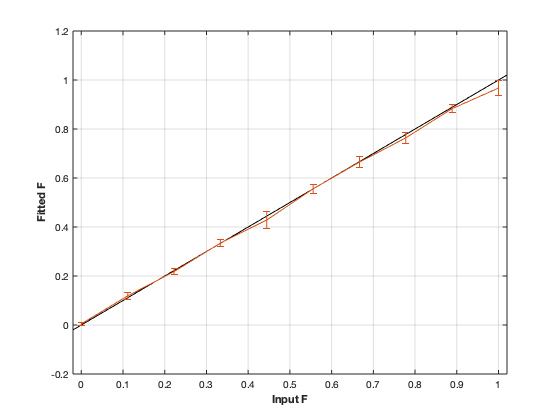# qmt_sirfse: qMT using Inversion Recovery Fast Spin Echo acquisition¶## Contents

```% This m-file has been automatically generated using qMRgenBatch(qmt_sirfse)
% Command Line Interface (CLI) is well-suited for automatization
% purposes and Octave.
%
% Please execute this m-file section by section to get familiar with batch
% processing for qmt_sirfse on CLI.
%
%
% Written by: Agah Karakuzu, 2017
% =========================================================================
```

## I- DESCRIPTION

```qMRinfo('qmt_sirfse'); % Describe the model
```
```  qmt_sirfse:  qMT using Inversion Recovery Fast Spin Echo acquisition
a href="matlab: figure, imshow qmt_sirfse.png ;"Pulse Sequence Diagram/a

ASSUMPTIONS:
(1) FILL
(2)
(3)
(4)

Inputs:
MTdata              Magnetization Transfert data
(R1map)             1/T1map (OPTIONAL but recommended)

Outputs:
F                   Ratio of number of restricted pool to free pool, defined
as F = M0r/M0f = kf/kr.
kr                  Exchange rate from the free to the restricted pool
(note that kf and kr are related to one another via the
definition of F. Changing the value of kf will change kr
accordingly, and vice versa).
R1f                 Longitudinal relaxation rate of the free pool
(R1f = 1/T1f).
R1r                 Longitudinal relaxation rate of the restricted pool
(R1r = 1/T1r).
Sf                  Instantaneous fraction of magnetization after vs. before
the pulse in the free pool. Starting point is computed using Block
simulation.
Sr                  Instantaneous fraction of magnetization after vs. before
the pulse in the restricted pool. Starting point is computed using block
simulation.
M0f                 Equilibrium value of the free pool longitudinal
magnetization.
(M0r)               Equilibrium value of the restricted pool longitudinal
magnetization. Computed using M0f = M0r * F.
(kf)                Exchange rate from the restricted to the free pool.
Computed using kf = kr * F.
(resnorm)           Fitting residual.

Protocol:
MTdata
Ti                Inversion times (s)
Td                Delay times (s)

FSEsequence
Trf               Duration of the pulses in the FSE sequence (s)
Tr                Delay between the pulses in the FSE sequnece (s)
Npulse            Number of refocusing pulses in the FSE sequence

Options:
Inversion Pulse
Shape             Shape of the inversion pulse.
Available shapes are:
- hard
- gaussian
- gausshann (gaussian pulse with Hanning window)
- sinc
- sinchann (sinc pulse with Hanning window)
- singauss (sinc pulse with gaussian window)
- fermi
Duration          Duration of the inversion pulse (s)

Fitting
Use R1map to      By checking this box, you tell the fitting
constrain R1f       algorithm to check for an observed R1map and use
its value to constrain R1f. Checking this box
will automatically set the R1f fix box to true in
the Fit parameters table.
Fix R1r = R1f     By checking this box, you tell the fitting
algorithm to fix R1r equal to R1f. Checking this
box will automatically set the R1r fix box to
true in the Fit parameters table.

Sr Calculation
Lineshape         The absorption lineshape of the restricted pool. Available lineshapes are: Gaussian, Lorentzian and SuperLorentzian.
T2r               Transverse relaxation time of the restricted pool (T2r = 1/R2r)

Example of command line usage:
For more examples: a href="matlab: qMRusage(qmt_sirfse);"qMRusage(qmt_sirfse)/a

Author: Ian Gagnon, 2017

Reference page in Doc Center
doc qmt_sirfse

```

## a- create object

```Model = qmt_sirfse;
```

## b- modify options

```         |- This section will pop-up the options GUI. Close window to continue.
|- Octave is not GUI compatible. Modify Model.options directly.```
```Model = Custom_OptionsGUI(Model); % You need to close GUI to move on.
```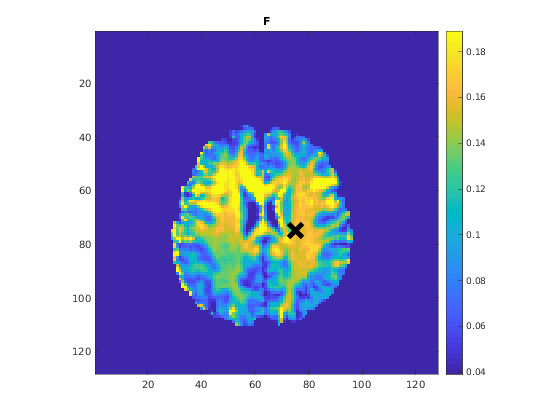## III- FIT EXPERIMENTAL DATASET

```         |- qmt_sirfse object needs 3 data input(s) to be assigned:
|-   MTdata
|-   R1map
```data = struct();
% MTdata.nii.gz contains [128  128    1   25] data.
% Mask.nii.gz contains [128  128] data.
```

## b- fit dataset

`           |- This section will fit data.`
```FitResults = FitData(data,Model,0);
```
```=============== qMRLab::Fit ======================
Operation has been started: qmt_sirfse
Elapsed time is 0.091363 seconds.
Operation has been completed: qmt_sirfse
==================================================
```

## c- show fitting results

```         |- Output map will be displayed.
|- If available, a graph will be displayed to show fitting in a voxel.
|- To make documentation generation and our CI tests faster for this model,
we used a subportion of the data (40X40X40) in our testing environment.
|- Therefore, this example will use FitResults that comes with OSF data for display purposes.
|- Users will get the whole dataset (384X336X224) and the script that uses it for demo
via qMRgenBatch(qsm_sb) command.```
```FitResults_old = load('FitResults/FitResults.mat');
qMRshowOutput(FitResults_old,data,Model);
```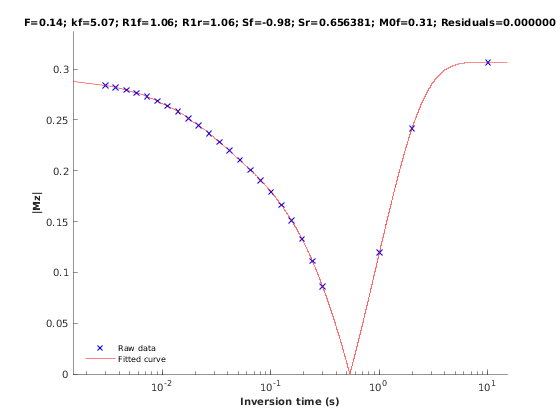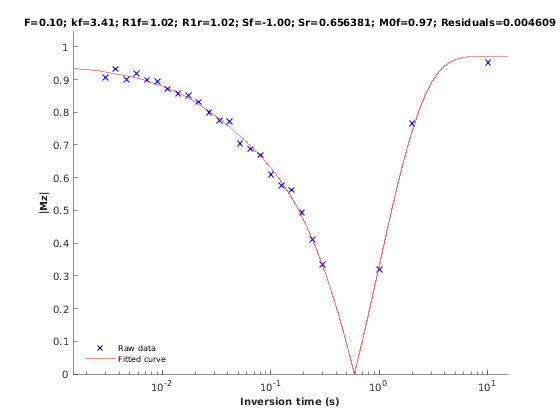## d- Save results

```         |-  qMR maps are saved in NIFTI and in a structure FitResults.mat
that can be loaded in qMRLab graphical user interface
|-  Model object stores all the options and protocol.
It can be easily shared with collaborators to fit their
own data or can be used for simulation.```
```FitResultsSave_nii(FitResults, 'qmt_sirfse_data/MTdata.nii.gz');
Model.saveObj('qmt_sirfse_Demo.qmrlab.mat');
```
```Warning: Directory already exists.
```

## V- SIMULATIONS

`   |- This section can be executed to run simulations for qmt_sirfse.`

## a- Single Voxel Curve

```         |- Simulates Single Voxel curves:
(1) use equation to generate synthetic MRI data
(3) fit and plot curve```
```      x = struct;
x.F = 0.1;
x.kr = 30;
x.R1f = 1;
x.R1r = 1;
x.Sf = -0.98419;
x.Sr = 0.65638;
x.M0f = 1;
% Set simulation options
Opt.SNR = 50;
Opt.Method = 'Analytical equation';
Opt.T2fUsedinBlockequation = 0.04;
% run simulation
figure('Name','Single Voxel Curve Simulation');
FitResult = Model.Sim_Single_Voxel_Curve(x,Opt);
```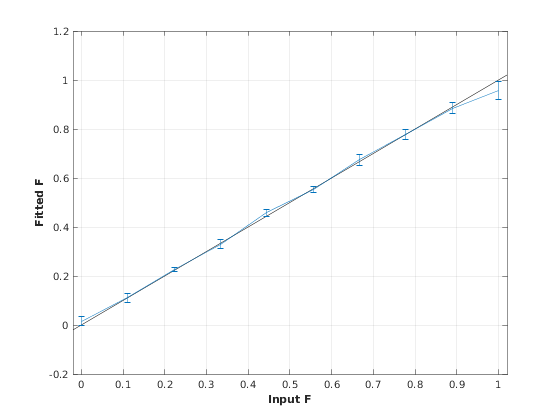## b- Sensitivity Analysis

```         |-    Simulates sensitivity to fitted parameters:
(1) vary fitting parameters from lower (lb) to upper (ub) bound.
(2) run Sim_Single_Voxel_Curve Nofruns times
(3) Compute mean and std across runs```
```      %              F             kr            R1f           R1r           Sf            Sr            M0f
OptTable.st = [0.1           30            1             1             -0.98         0.66          1]; % nominal values
OptTable.fx = [0             1             1             1             1             1             1]; %vary F...
OptTable.lb = [0.0001        0.0001        0.05          0.05          -1            0.0001        0.0001]; %...from 0.0001
OptTable.ub = [1             1e+02         10            10            0             1             2]; %...to 1
% Set simulation options
Opt.SNR = 50;
Opt.Method = 'Analytical equation';
Opt.T2fUsedinBlockequation = 0.04;
Opt.Nofrun = 5;
% run simulation
SimResults = Model.Sim_Sensitivity_Analysis(OptTable,Opt);
figure('Name','Sensitivity Analysis');
SimVaryPlot(SimResults, 'F' ,'F' );
```